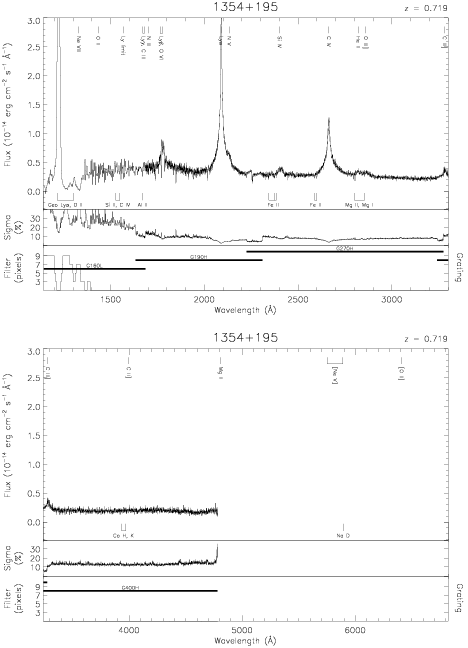Download dataset
TELESCOP= 'HST     '
INSTRUME= 'FOS     '
EQUINOX =               2000.0
DATE-OBS= '1992-02-26T19:52:13'
RA_PNT  =       209.2687376924
DEC_PNT =        19.3184958975
PA_PNT  =        48.3807327322
OBJECT  = '1354+195'
ROOTNM01= 'Y0RV1303T'
DETECT01= 'AMBER   '
APERID01= 'C-2     '
FGWAID01= 'H19     '
ROOTNM02= 'Y0RV1304T'
DETECT02= 'AMBER   '
APERID02= 'C-2     '
FGWAID02= 'H19     '
ROOTNM03= 'Y0RV1305T'
DETECT03= 'AMBER   '
APERID03= 'C-2     '
FGWAID03= 'H27     '
ROOTNM04= 'Y0RV1306T'
DETECT04= 'BLUE    '
APERID04= 'B-3     '
FGWAID04= 'L15     '
ROOTNM05= 'Y12B0802T'
GRNDMD05= 'SPECTROSCOPY'
DETECT05= 'AMBER   '
APERID05= 'A-1     '
FGWAID05= 'H19     '
ROOTNM06= 'Y12B0803T'
GRNDMD06= 'SPECTROSCOPY'
DETECT06= 'AMBER   '
APERID06= 'A-1     '
FGWAID06= 'H27     '
ROOTNM07= 'Y12B0804T'
GRNDMD07= 'SPECTROSCOPY'
DETECT07= 'AMBER   '
APERID07= 'A-1     '
FGWAID07= 'H40     '